Members Area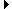Sign Up | Log In
 Free Level 2 QuotesStock AnalysisCorrelation Trading PlatformCorrelationsMembership Benefits

AVEVOL
 Lookup a different indicator.

 Indicator Usage Description Example avevol avevol[x,n] OPERATOR (INDICATOR or POSITIVE NUMBER) , where x is a positive integer >= 2 and n is optional and represents the number of days ago the result is computed (n=0 is the default value and represents the current end of day, n=1 is the previous end of day, etc.) Returns the x-day simple moving average volume from n days ago. (avevol < (0.8 * avevol))

Introduction:

The 'avevol' indicator is used in indicator formula construction to narrow the stock pre-screener results to include only those stocks that have a new high stock price restricted to the parameters set for the avevol indicator.

Example:

The chart of Intel (INTC) below uses the example indicator above to highlight days where the 5-day average volume was less than 80% of the 50-day average volume.

 Chart of Intel (INTC) highlighting days where the 5-day average volume was less than 80% of the 50-day average volume.

INDICATORS IN OUR DATABASE THAT INCLUDE THE AVEVOL INDICATOR...

Click here to view all indicators that include the indicator 'avevol' (sorted by AISCORE)

 (vol > 1.5 * avevol) and (close > sma)AISCORE: 1492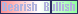Indicator ID: 051102155300(vol > 1.5 * avevol) and (close < (0.95 * sma))AISCORE: 4054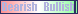Indicator ID: 051212205209(vol > 1.5 * avevol) and (close < (0.98 * sma)) and (close < (0.98 * sma))AISCORE: 4722Indicator ID: 051212205358(vol > 1.85 * avevol) and (close < (0.97 * sma))AISCORE: 171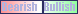Indicator ID: 051219224302(vol > 1.5 * avevol) and (vol > 1.5 * avevol) and (close < (0.98 * sma))AISCORE: 3525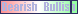Indicator ID: 051222183746 More ->